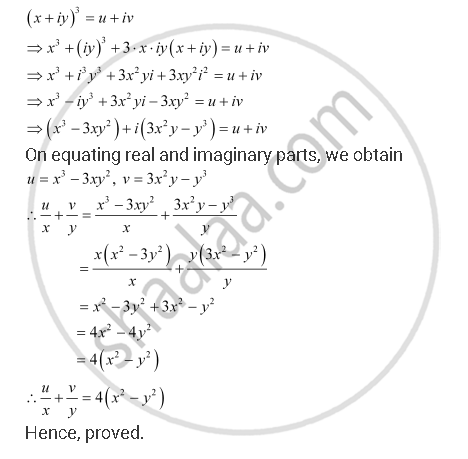CBSE (Arts) Class 11CBSE
Share

# If (X + Iy)3 = U + Iv, Then Show that U/X + V/Y =4(X^2 - Y^2) - CBSE (Arts) Class 11 - Mathematics

ConceptThe Modulus and the Conjugate of a Complex Number

#### Question

If (x + iy)3 = u + iv, then show that u/x + v/y  =4(x^2 - y^2)

#### SolutionIs there an error in this question or solution?

#### Video TutorialsVIEW ALL 

Solution If (X + Iy)3 = U + Iv, Then Show that U/X + V/Y =4(X^2 - Y^2) Concept: The Modulus and the Conjugate of a Complex Number.
S